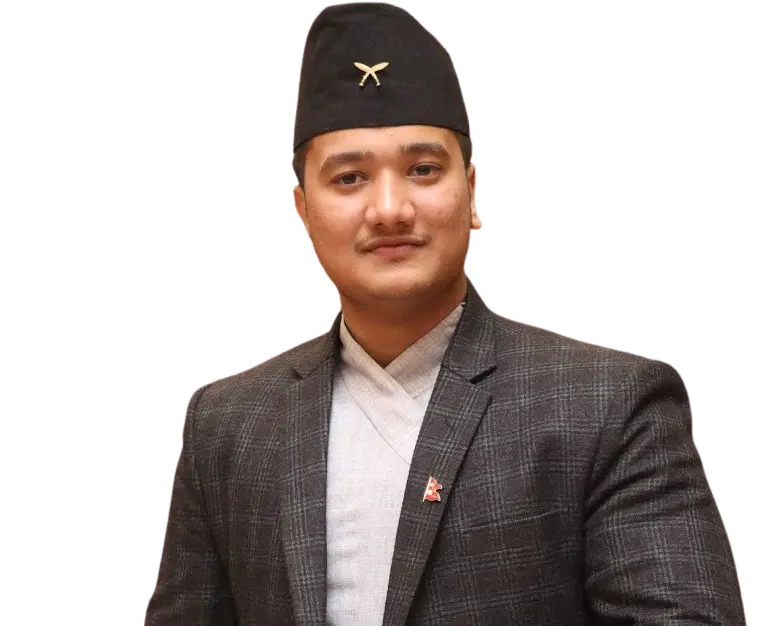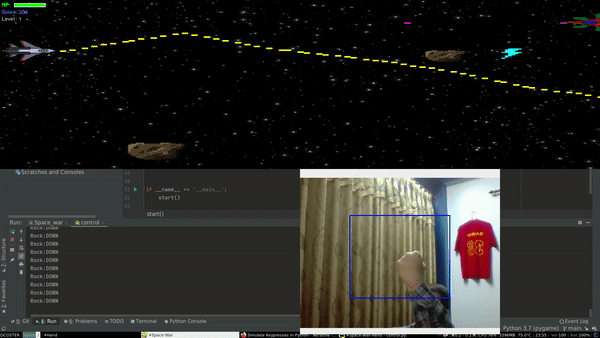# Make Your Own AI Powered Gameby Sabin

## Gaming with a keyboard is fun, but using your webcam makes it even better.Project Demo

As usual, we will apply the divide-and-conquer concept to create our system. Basically, we will need to break down our dilemma (create an AI-powered game) into lighter sub-obstacles. I got up with the successive obstacle set:

1. We need a game
2. Since we are accepting a webcam, we require a medium that facilitates us to pick up our hand movements
3. After we pick up our hand actions, we require an instrument that labels the actions into some sort of logic
4. Last, we require a controller that regulates the comprehensive actions of the game from the logic developed

You can find the source code below:

So first we need a game to get started. After a few minutes of wondering, I came up with an elite weapon — “copy & paste”. It’s better to use someone else’s code than writing it from scratch. So I did the same, went to pygames.org, browsed through some webpages, and came up with a game called Space War. Since I was not planning to use complex movements within the game, Space war fit’s the requirement just perfectly. It has basic actions like move up, down, forward, backward and shoot.

Now we have a game to play. Next thing we need is to get our hand movements from our webcam. Since I had some experience with facial recognition, I was pretty familiar with OpenCV which will help us to capture hand gestures. Adding up till now we have a game and a subsystem to get our hand movements.

Similarly, We need a mechanism that converts our hand movements into some sort of logic. As I was getting familiar with computer vision, I made a classifier that classifies an image into 3 distinct classes i.e. Rock , Paper and Scissor using convolution neural networks(CNN’s). For simplicity, I will use only two of this logic.

Rock -> move down

Paper ->move up

Last, we require a controller. After performing some exploration (googling) I got up with an awesome python library called pynput from which we can replicate keyboard activities from our system. All I require now is to unite explanations of these different sub processes into one full performing structure.

So I wrote a script that merges solution of our four problems:

``````import cv2
import os
import tensorflow as tf
import numpy as np
from pynput.keyboard import Key, Controller                                               c = Key.space
monitor_height = 1080
monitor_width = 1920
keyboard = Controller()
def start():
cap = cv2.VideoCapture(0)
while True:

keyboard.press(Key.space)
predict_image = img.copy()
predict_image = cv2.flip(predict_image, 1)
height, width = predict_image.shape[:2]
x1, y1 = int(width * 0.25), int(height * 0.25)
x2, y2 = int(width * 0.75), int(height * 0.8)
cv2.rectangle(img, (x1, y1), (x2, y2), (255, 0, 0), 2)
predict_image = img[y1:y2, x1:x2]
cv2.imshow("Hand", cv2.flip(img, 1))
cv2.moveWindow("Hand", int(monitor_width / 2), int(monitor_height / 2))
predict_image = cv2.resize(predict_image, (150, 150), interpolation=cv2.INTER_AREA)
cv2.imwrite("a.png", predict_image)
predict_image =
tf.keras.preprocessing.image.img_to_array(predict_image)
predict_image = np.expand_dims(predict_image / 2, axis=0)
prediction = model.predict(predict_image)
if prediction == 1:
c = Key.up
print("Paper:UP")
elif prediction == 1:
c = Key.down
print("Rock:DOWN")
keyboard.release(Key.space)
keyboard.press(c)
key = cv2.waitKey(60)
if key == 27:
break
keyboard.release(c)
keyboard.release(c)
cap.release()
cv2.destroyAllWindows()
if __name__ == '__main__':
start()
``````

Code Explanation :

``````cap = cv2.VideoCapture(0)
``````

load our per-trained model that classifies images into 3 classes

``````model = tf.keras.models.load_model("Model/rps2.h5")
``````

Capture and predict the images:

``````while True:
// reading the image from the web cam

keyboard.press(Key.space)
// creating a copy of the image received
predict_image = img.copy()
// flipping the image for the right orientation
predict_image = cv2.flip(predict_image, 1)
// getting the height and width of the image
height, width = predict_image.shape[:2]
// defining the coordinates for bounding boxes
x1, y1 = int(width * 0.25), int(height * 0.25)
x2, y2 = int(width * 0.75), int(height * 0.8)
// creating a bounding box to place our hand
cv2.rectangle(img, (x1, y1), (x2, y2), (255, 0, 0), 2)
// getting the image within the bounding box
predict_image = img[y1:y2, x1:x2]
cv2.imshow("Hand", cv2.flip(img, 1))
cv2.moveWindow("Hand", int(monitor_width / 2), int(monitor_height / 2))
// resizing the image so that it matches out NN's input
predict_image = cv2.resize(predict_image, (150, 150), interpolation=cv2.INTER_AREA)
// writing the image into the disk
cv2.imwrite("a.png", predict_image)
// converting the image into an array
predict_image =
tf.keras.preprocessing.image.img_to_array(predict_image)
// adding dimension since NN's takes input of 4 dims
predict_image = np.expand_dims(predict_image / 2, axis=0)
// predicting the image
prediction = model.predict(predict_image)
``````

Perform action according to the prediction:

``````if prediction == 1:
// move up by pressing up arrow if it is paper
c = Key.up
print("Paper:UP")
elif prediction == 1:
// move down by pressing down arrow if it is rock
c = Key.down
print("Rock:DOWN")
// for triggering shooting action
keyboard.release(Key.space)
// releasing the key after key press
keyboard.press(c)
// wait if the user want's to quit
key = cv2.waitKey(60)
if key == 27:
break
keyboard.release(c)
``````

So at last, all we need to do is fire up the game along with this script.

To learn how to run this game, go through the above specified GitHub repo or visit here. Now we have a game that can be played from a webcam with AI sprayed on top!